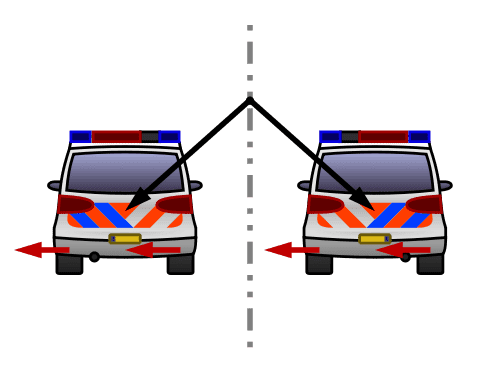# Direction of Pseudovectors

• SgrA*
In summary, the Wikipedia article on pseudovectors initially suggests that they change direction after reflection. However, this is not always the case as some physical examples, such as angular momentum, remain invariant under reflection. The article also discusses the concept of pseudovectors being direction invariant under reflection, but this statement is not entirely accurate as reflecting in a mirror parallel to the vector can result in a change in sign. Overall, the article's explanation of pseudovectors and their behavior under reflection is confusing and may require further clarification.

#### SgrA*

The Wikipedia article on http://en.wikipedia.org/wiki/Pseudovector" [Broken] initally suggests that they do change direction after reflection.

Geometrically it (a pseudovector) is the opposite, of equal magnitude but in the opposite direction, of its mirror image. This is as opposed to a true or polar vector (more formally, a contravariant vector), which on reflection matches its mirror image.

But in a later http://en.wikipedia.org/wiki/Pseudovector#Physical_examples" angular momentum remains invariant under reflection.

Each wheel of a car driving away from an observer has an angular momentum pseudovector pointing left. The same is true for the mirror image of the car.

A pseudovector is direction invariant under reflection or not?

I tried to devise a trick on the Right Hand Rule - if I were to switch to a left hand rule (left hand appears to be a mirror image of the right hand), and curl my left palm as in right hand rule. I noticed that if the left hand is to be maintained as a mirror image of the right, the direction of both the thumbs is same, suggesting that it is invariant.

Last edited by a moderator:
SgrA* said:
The Wikipedia article on http://en.wikipedia.org/wiki/Pseudovector" [Broken] initally suggests that they do change direction after reflection.
Geometrically it (a pseudovector) is the opposite, of equal magnitude but in the opposite direction, of its mirror image. This is as opposed to a true or polar vector (more formally, a contravariant vector), which on reflection matches its mirror image.
But in a later http://en.wikipedia.org/wiki/Pseudovector#Physical_examples" angular momentum remains invariant under reflection.

Well, that's wikipedia for ya'. Sometimes very good, sometimes poorly written and confusing, sometimes flat out wrong. This article falls in the second category.Think of the car on the left as the real car, the car on the right as the car's reflection. Imagine a vector on the car's rear bumper, with the tail in the middle of the red/gray hatched region and the head in the middle of the red/blue hatched region. In the car on the left, this vector is parallel to the angular momentum vectors of the car's wheels. Upon reflection, this vector reverses direction: It is points to the right upon reflection. This displacement vector is a real vector rather than a pseudovector. It's reflected image is the reverse of the real image in this particular example. The angular momentum pseudovectors are reflected and reversed. Reflection alone would reverse the direction of the pseudovectors. Reversing the reflections of these pseudovectors leaves those pseudovectors unchanged in this particular example.

Last edited by a moderator:
Actually, the original statement is not complete and not correct. It is true that a pseudovector, reflected in a mirror perpendicular to the vector, does not change sign. If you reflect a pseudovector in a mirror parallel to the vector, it does change sign.

You misread that statement in the wikipedia article. The statement is correct, just very poorly worded.

## 1. What is the direction of a pseudovector?

A pseudovector is a type of vector that has a magnitude and direction, but its direction is opposite to what would be expected based on its physical properties. This means that the direction of a pseudovector can be determined by considering the properties of the system it is acting on.

## 2. How is the direction of a pseudovector different from a regular vector?

Unlike regular vectors, which follow the right-hand rule for determining direction, pseudovectors follow the left-hand rule. This means that if you were to curl the fingers of your left hand in the direction of the vector, your thumb would point in the opposite direction, which is the direction of the pseudovector.

## 3. What are some examples of pseudovectors?

Some common examples of pseudovectors include angular velocity, magnetic field, and torque. These vectors have a direction that is opposite to what would be expected based on their physical properties.

## 4. How does the direction of a pseudovector affect its behavior?

The direction of a pseudovector is an important factor in determining its behavior. For example, the direction of a magnetic field affects how charged particles will move through it, and the direction of torque affects how an object will rotate.

## 5. Can the direction of a pseudovector be changed?

No, the direction of a pseudovector is determined by the properties of the system it is acting on and cannot be changed. However, the magnitude of the vector can be altered by changing the physical properties of the system.